## Prediction Tutorial¶

Simulates "true data" corrected by acceptance, according to\ the fitted values for the amplitude

In :
import PyPWA as pwa
import numpy as npy
import pandas
import matplotlib.pyplot as plt
import warnings
warnings.filterwarnings('ignore')


# Define waves and amplitude (function) to simulate¶

In :
initial=[]
for param_type in ["r", "i"]:
initial.append(f"{param_type}.1.0.0")
initial.append(f"{param_type}.1.1.0")
initial.append(f"{param_type}.1.1.1")
initial.append(f"{param_type}.1.2.0")
initial.append(f"{param_type}.1.2.1")
initial.append(f"{param_type}.1.2.2")
#import AmplitudeOLDfit
#amp = AmplitudeOLDfit.FitAmplitude(initial)
#
import AmplitudeJPACsim
amp = AmplitudeJPACsim.NewAmplitude()
#
#import AmplitudeJPACfit
#amp = AmplitudeJPACfit.FitAmplitude(initial)


# Read input (flat) simulated (generated) data¶

In :
data = pwa.read("etapiHEL2_flat.txt")


Read flat data in gamp format (full information)

In :
datag = pwa.read("../TUTORIAL_FILES/raw_events.gamp")


Define number of bins (MUST be the same as the fitted parameters)

In :
nbins=20
bins = pwa.bin_by_range(data, "mass", nbins, .6, 2.0)


Calculate the mass value and number of events in each bin

In :
bmass=[]
mcounts=[]
for index, bin in enumerate(bins):
if len(bin)==0:
bmass.append(0.)
mcounts.append(0.)
else:
bmass.append(npy.average(bin["mass"]))
mcounts.append(len(bin))


# Read parameters from fit¶

In :
par = pwa.read("final_values_JPAC.csv")


Prepare for a binned simulation

Find intensities in each bin and max intensity for each bin.

In :
int_values = []
max_values = []
params={}
for index, bin in enumerate(bins):
for param_type in ["r", "i"]:
params.update({f"{param_type}.1.0.0":par[f"{param_type}.1.0.0"][index]})
params.update({f"{param_type}.1.1.0":par[f"{param_type}.1.1.0"][index]})
params.update({f"{param_type}.1.1.1":par[f"{param_type}.1.1.1"][index]})
params.update({f"{param_type}.1.2.0":par[f"{param_type}.1.2.0"][index]})
params.update({f"{param_type}.1.2.1":par[f"{param_type}.1.2.1"][index]})
params.update({f"{param_type}.1.2.2":par[f"{param_type}.1.2.2"][index]})

[int,intmax] = pwa.simulate.process_user_function(amp,bin,params,16)
int_values.append(int)
max_values.append(intmax)


# Simulate events for each bin (produce mask and mask them)¶

In :
rejected_bins = []
for int_value, bin in zip(int_values, bins):
rejection = pwa.simulate.make_rejection_list(int_value, max_values)
rejected_bins.append(bin[rejection])


Check how many events simulated by bin

In :
for index, simulated_bin in enumerate(rejected_bins):
print(
f"Bin {index+1}'s length is {len(simulated_bin)}, "
f"{(len(simulated_bin) / len(bin)) * 100:.2f}% events were kept"
)

Bin 1's length is 4956, 1.83% events were kept
Bin 2's length is 6431, 2.38% events were kept
Bin 3's length is 10409, 3.84% events were kept
Bin 4's length is 24952, 9.22% events were kept
Bin 5's length is 66596, 24.60% events were kept
Bin 6's length is 27353, 10.10% events were kept
Bin 7's length is 15198, 5.61% events were kept
Bin 8's length is 17851, 6.59% events were kept
Bin 9's length is 31845, 11.76% events were kept
Bin 10's length is 45846, 16.93% events were kept
Bin 11's length is 20052, 7.41% events were kept
Bin 12's length is 10672, 3.94% events were kept
Bin 13's length is 10547, 3.90% events were kept
Bin 14's length is 12380, 4.57% events were kept
Bin 15's length is 15457, 5.71% events were kept
Bin 16's length is 18446, 6.81% events were kept
Bin 17's length is 17660, 6.52% events were kept
Bin 18's length is 12511, 4.62% events were kept
Bin 19's length is 8275, 3.06% events were kept
Bin 20's length is 5700, 2.11% events were kept


Stak data for all bins in one file (new_data)

In :
for index, the_bin in zip(range(len(rejected_bins)), rejected_bins):
if index ==0:
new_data=pandas.DataFrame(the_bin)

new_data =  new_data.append(the_bin,ignore_index=True)

#print(new_data)

In :
new_data

Out:
EventN theta phi alpha pol tM mass
0 2489.0 2.741230 4.72455 2.262170 0.4 -0.006800 0.726344
1 3015.0 1.747350 3.00551 2.200170 0.4 -0.229975 0.691760
2 3378.0 1.422730 4.79784 -0.898373 0.4 -0.607336 0.737398
3 8067.0 0.799254 3.20964 -2.552260 0.4 -0.062793 0.703809
4 9098.0 0.518186 4.58191 -2.107370 0.4 -0.152568 0.736795
... ... ... ... ... ... ... ...
388088 9842238.0 1.558980 4.48915 -1.294070 0.4 -0.775586 1.984570
388089 9842471.0 0.619778 3.40782 -0.278216 0.4 -0.186332 1.947310
388090 9845141.0 0.727831 1.27644 1.663220 0.4 -0.496527 1.964230
388091 9847305.0 1.061280 2.20065 -0.438635 0.4 -0.114632 1.990740
388092 9851949.0 0.919222 4.29291 -2.161100 0.4 -0.080871 1.994540

388093 rows × 7 columns

# Calculate the number of expected events versus mass bins¶

In :
total_nExp = npy.empty(len(rejected_bins))
for index, the_bin in zip(range(len(rejected_bins)), rejected_bins):

for param_type in ["r", "i"]:
params.update({f"{param_type}.1.0.0":par[f"{param_type}.1.0.0"][index]})
params.update({f"{param_type}.1.1.0":par[f"{param_type}.1.1.0"][index]})
params.update({f"{param_type}.1.1.1":par[f"{param_type}.1.1.1"][index]})
params.update({f"{param_type}.1.2.0":par[f"{param_type}.1.2.0"][index]})
params.update({f"{param_type}.1.2.1":par[f"{param_type}.1.2.1"][index]})
params.update({f"{param_type}.1.2.2":par[f"{param_type}.1.2.2"][index]})
amp.setup(the_bin)
total_nExp[index] = npy.average(amp.calculate(params))


Plot number of true (100% acc) events versus mass

In :
mni = npy.empty(len(total_nExp), dtype=[("mass", float), ("int", float)])
mni["mass"] = bmass
mni["int"] = total_nExp
mni = pandas.DataFrame(mni)
counts, bin_edges = npy.histogram(mni["mass"], nbins, weights=mni["int"])

centers = (bin_edges[:-1] + bin_edges[1:]) / 2

# Add yerr to argment list when we have errors
yerr = npy.empty(nbins)
yerr = npy.sqrt(counts)
yerr=0.
plt.errorbar(centers,counts, yerr, fmt="s",linestyle="dashed",markersize='10',label="nExp")

#plt.xlim(.6, 3.2)
#plt.ylim(0.,65000)
plt.legend(loc='upper right')
plt.show()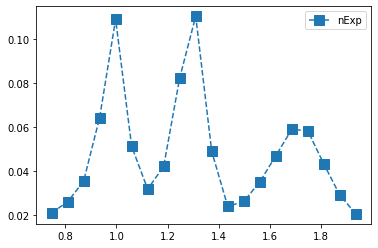# Calculate Phase difference between two waves¶

In :
V1=npy.ndarray(len(par))
V2=npy.ndarray(len(par))
V1 = par["r.1.1.1"]+par["i.1.1.1"]*(1j)
V2 = par["r.1.2.1"]+par["i.1.2.1"]*(1j)
if V1.all() != 0 and V2.all() != 0:
phasediff = npy.arctan(npy.imag(V1*npy.conj(V2))/npy.real(V1*npy.conj(V2)))


Plot PhaseMotion

In :
mnip = npy.empty(len(bins), dtype=[("mass", float), ("phase", float)])
mnip["mass"] = bmass
mnip["phase"] = phasediff
mnip = pandas.DataFrame(mnip)
counts, bin_edges = npy.histogram(mnip["mass"], 25, weights=mnip["phase"])
centers = (bin_edges[:-1] + bin_edges[1:]) / 2

# Add yerr to argment list when we have errors
yerr = npy.empty(100)
yerr = npy.sqrt(npy.abs(counts))
plt.errorbar(centers,counts, yerr, fmt="o")
plt.xlim(0.6, 3.2)

Out:
(0.6, 3.2)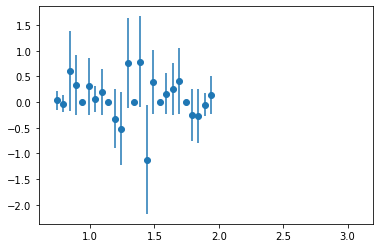Plot phi_HEL vs cos(theta_HEL) of predicted data (with 4 different contracts(gamma))

In :
import matplotlib.colors as mcolors
from numpy.random import multivariate_normal

gammas = [0.8, 0.5, 0.3]

fig, axes = plt.subplots(nrows=2, ncols=2)

axes[0, 0].set_title('Linear normalization')
axes[0, 0].hist2d(new_data["phi"], npy.cos(new_data["theta"]), bins=100)
#axes[0, 0].hist2d(cut_list["phi"], npy.cos(cut_list["theta"]), bins=100)

for ax, gamma in zip(axes.flat[1:], gammas):
ax.set_title(r'Power law $(\gamma=%1.1f)$' % gamma)
ax.hist2d(new_data["phi"], npy.cos(new_data["theta"]),
bins=100, norm=mcolors.PowerNorm(gamma))

fig.tight_layout()

plt.show()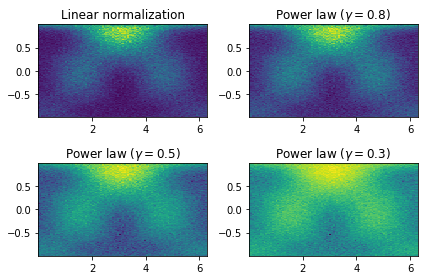Plot cos(theta_HEL) vs mass for simulated data (with 4 different contrasts)

In :
gammas = [0.8, 0.5, 0.3]

fig, axes = plt.subplots(nrows=2, ncols=2)

axes[0, 0].set_title('Linear normalization')
axes[0, 0].hist2d(new_data["mass"], npy.cos(new_data["theta"]),bins=100)

for ax, gamma in zip(axes.flat[1:], gammas):
ax.set_title(r'Power law $(\gamma=%1.1f)$' % gamma)
ax.hist2d(new_data["mass"], npy.cos(new_data["theta"]),
bins=100, norm=mcolors.PowerNorm(gamma))

fig.tight_layout()
plt.xlim(.6, 2.)
plt.show()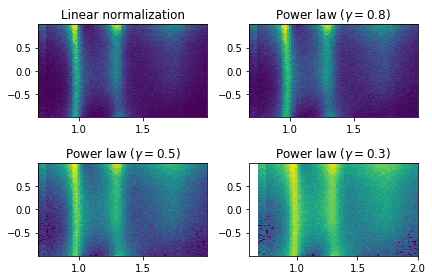Plot phi_HEL vs mass for simulated data (with 4 different contrasts)

In :
gammas = [0.8, 0.5, 0.3]

fig, axes = plt.subplots(nrows=2, ncols=2)

axes[0, 0].set_title('Linear normalization')
axes[0, 0].hist2d(new_data["mass"], new_data["phi"],bins=100)

for ax, gamma in zip(axes.flat[1:], gammas):
ax.set_title(r'Power law $(\gamma=%1.1f)$' % gamma)
ax.hist2d(new_data["mass"], new_data["phi"],
bins=100, norm=mcolors.PowerNorm(gamma))

fig.tight_layout()
plt.xlim(.6, 2.)
plt.show()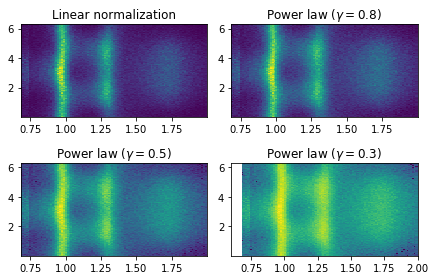In [ ]:


In :
plt.hist(new_data["alpha"],50)
plt.show()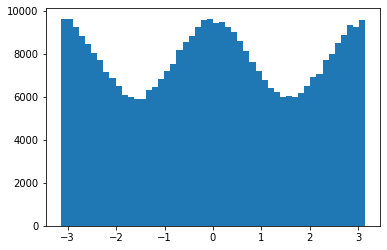Plot mass versus alpha/Phi

In :
gammas = [0.8, 0.5, 0.3]

fig, axes = plt.subplots(nrows=2, ncols=2)

axes[0, 0].set_title('Linear normalization')
axes[0, 0].hist2d(new_data["mass"], new_data["alpha"],bins=100)

for ax, gamma in zip(axes.flat[1:], gammas):
ax.set_title(r'Power law $(\gamma=%1.1f)$' % gamma)
ax.hist2d(new_data["mass"], new_data["alpha"],
bins=100, norm=mcolors.PowerNorm(gamma))

fig.tight_layout()
plt.xlim(.6, 2.)
plt.show()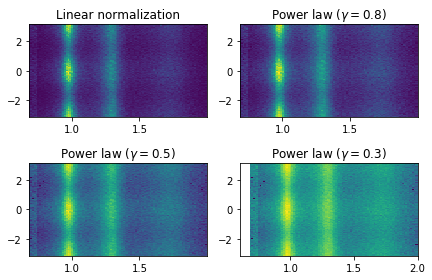Plot mass versus alpha/Phi

In :
gammas = [0.8, 0.5, 0.3]

fig, axes = plt.subplots(nrows=2, ncols=2)

axes[0, 0].set_title('Linear normalization')
axes[0, 0].hist2d(new_data["phi"],new_data["alpha"],bins=100)

for ax, gamma in zip(axes.flat[1:], gammas):
ax.set_title(r'Power law $(\gamma=%1.1f)$' % gamma)
ax.hist2d(new_data["phi"], new_data["alpha"],
bins=100, norm=mcolors.PowerNorm(gamma))

fig.tight_layout()

plt.show()